Function Repository Resource:

# LogDifferences

Find logarithmic differences of elements in a list

Contributed by: Stephen Wolfram
 ResourceFunction["LogDifferences"][list] gives the logarithmic differences of elements in list.

## Details and Options

ResourceFunction["LogDifferences"][list] for a list with elements of the form np is equivalent to ConstantArray[p,Length[list]-1].

## Examples

### Basic Examples (1)

Log differences for a length-n list of powers gives a list with n-1 copies of the exponent:

 In:=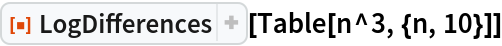Out=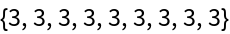### Scope (1)

Use a symbolic list:

 In:=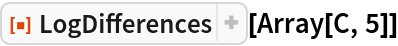Out=### Applications (3)

Find the connectivity graph for a Sierpiński mesh:

 In:=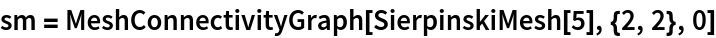Out=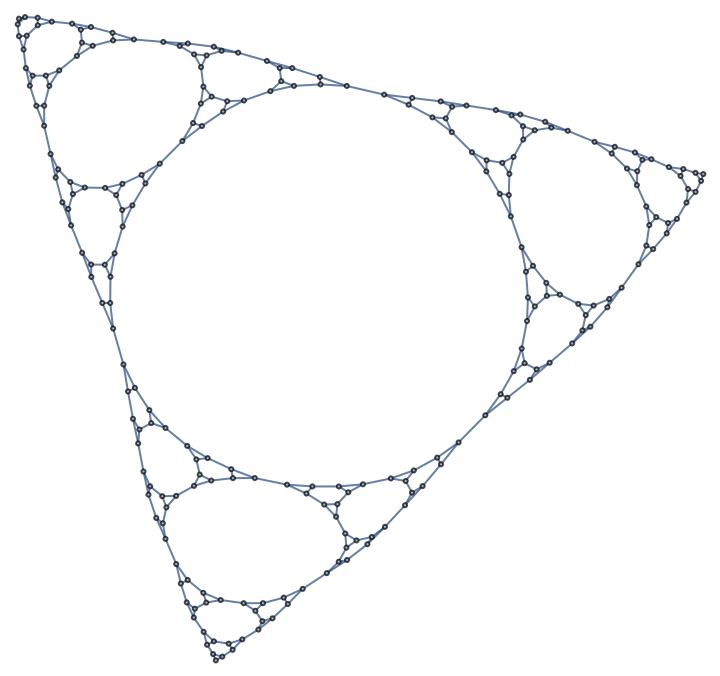Find successive average graph neighborhood volumes with the resource function GraphNeighborhoodVolumes:

 In:=Out=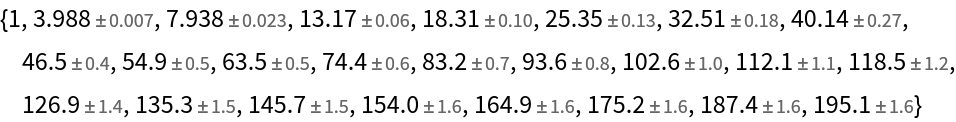Plot the log differences, which show an effective dimension around 1.58:

 In:=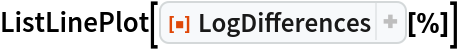Out=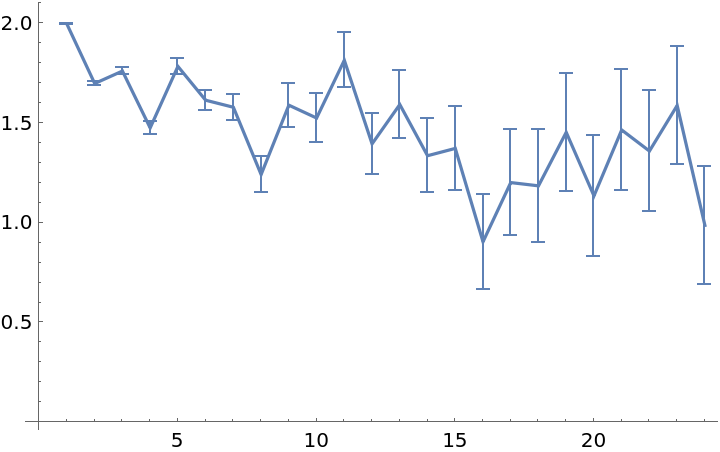## Version History

• 2.0.0 – 24 March 2020
• 1.0.0 – 04 December 2019